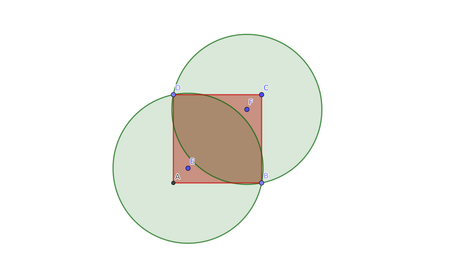# Network ReachrebornCriterion 2022 Round 19
Limits 1s, 256 MB

Shohurephone, the popular telecom company in Swapnodesh has received a lot of backlash over the last few years for not providing any coverage in the remote areas of the country. Adding insult to injury, the CEO himself got agitated yesterday when the CEO of their rival company, Kobi Ltd, called him from the remote areas of Monkeytown with a Kobi sim and said “Whazzup?” among many things. Thus, Shohurephone is now trying to enlarge their network coverage so that it covers the whole country.

Shohurephone has $n$ cell towers spread throughout the country. Installing a new tower is very costly and so, they won’t install any new ones. Instead, they will upgrade their antennas placed in the towers. The network setup in a tower usually covers a circular area of an uniform radius centered at the tower. Let’s define “Network Reach” of a tower to be this radius.

Shohurephone wants to upgrade the network reach of every tower, so that, together they cover all of the country. Additionally, they want their towers to have equal network reach as well for consistency. Upgrading the network reach by any margin is quite costly and they would like to minimize the cost. Calculate the minimum network reach needed for the towers so that together they provide network coverage to each part in the country.

We can describe Swapnodesh to be a convex polygon $P$ of $m$ points on a Cartesian grid. The $i^{th}$ vertex is at point $(a_i, b_i)$. The $n$ towers are located within this region, $i^{th}$ tower being at point $(x_i, y_i)$.

## Input

The first line of the input is an integer $T$ which denotes the number of test cases.

For each test case, the first line contains an integer $m$, denoting the number of vertices of the polygon $P$. $m$ lines follow, $i^{th}$ line containing two integers $a_i, b_i$ denoting the $i^{th}$ vertex. The vertices are given in a counter-clockwise order.

Another line follows, containing an integer, $n$, the number of towers. $n$ lines follow, $i^{th}$ line containing two integers $x_i, y_i$.

In all of the input files:

• $1 \le T \le 10^3$.

• $3 \le m \le 10^3$.

• $1 \le n \le 10^3$.

• $-10^9 \le a_i, b_i, x_i, y_i \le 10^9$.

• $\sum n$ and $\sum m$ over all test cases $\le 10^3$.

## Output

For each test case, output the minimum network reach needed for the towers on a single line.

Your output will be considered correct if the absolute or relative difference from the correct result is less than or equal to $10^{-6}$.

## Sample

InputOutput
1
4
0 0
6 0
6 6
0 6
2
1 1
5 5

5.0990195136ABCD (in red) is the polygonal shape of the country and E, F are the two towers. They have network coverage all over the green areas, thus covering the country.

### Submit